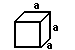Cube
 The volume of a cube is (side)^3. A Cube is a Regular Rectangular Prism. So it is arectagular prism with all congruent sides so we plugin in side under the apropriate postion in the formula (height)(length)(depth), which is the formula for the voume of rectangular prism. So the Volume of a cube will be side^3.